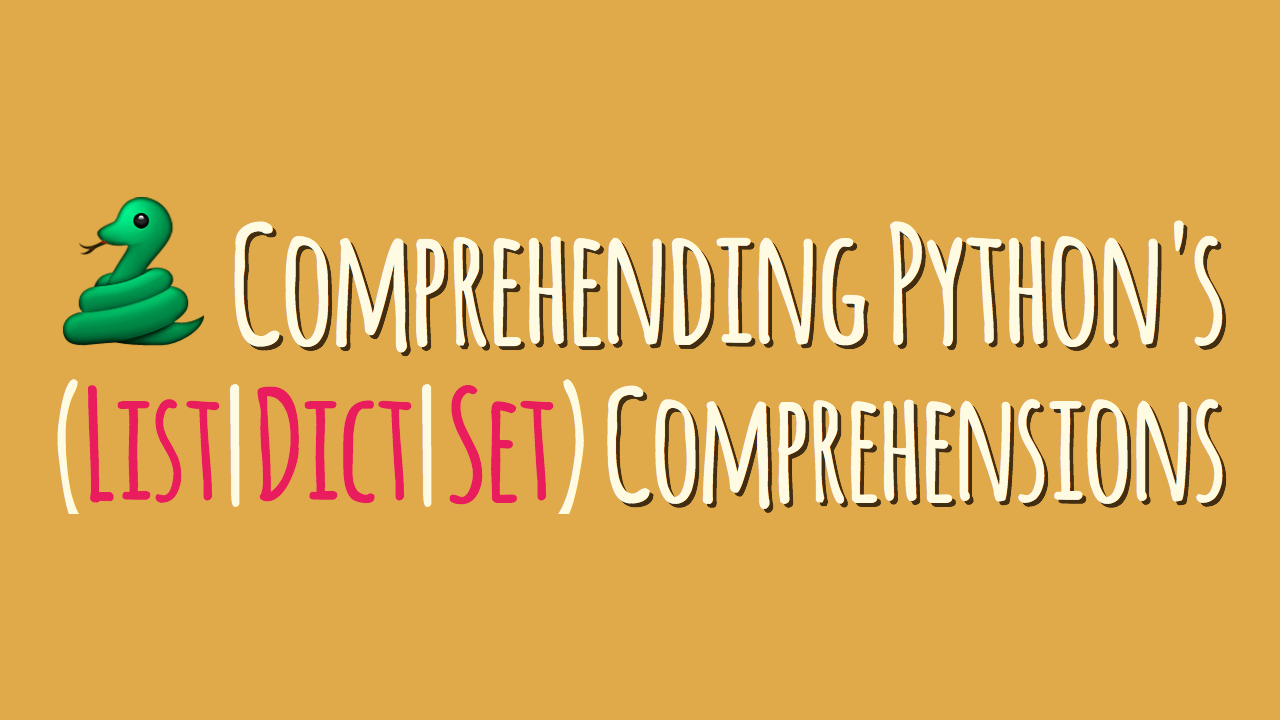Python List or any Iterable Comprehension

Sometimes a programming design pattern becomes common enough to warrant its own special syntax. Python’s list comprehensions are a prime example of such a syntactic sugar.

List comprehensions in Python are great, but mastering them can be tricky because they don’t solve a new problem: they just provide a new syntax to solve an existing problem.

Let’s learn what list comprehensions are and how to identify when to use them.

What are list comprehensions?

List comprehensions are a tool for transforming one list (any iterable actually) into another list. During this transformation, elements can be conditionally included in the new list and each element can be transformed as needed. An iterable is something you can loop over.

If you’re familiar with functional programming, you can think of list comprehensions as syntactic sugar for a filter followed by a map:

Unconditional Comprehensions

The components of a list comprehension are:

• Output Expression (Optional)
• Iterable
• Iterator variable which represents the members of the iterableExample:

We have a function:

squares = []
numbers = [1, 2, 3, 4, 5, 6, 7, 8, 9, 10]
for number in numbers:
squares.append(number ** 2)

print(squares)
[1, 4, 9, 16, 25, 36, 49, 64, 81, 100]

That same code written as a comprehension:

squares = [number ** 2 for number in numbers]

List Comprehensions with if statementWe can also create more advanced list comprehensions which include a conditional statement on the iterable.

squares = []
numbers = [1, 2, 3, 4, 5, 6, 7, 8, 9, 10]
for number in numbers:
if number % 2 == 0:
squares.append(number ** 2)

print(squares)
[4, 16, 36, 64, 100]

That same code written as a comprehension:

squares = [number ** 2  for number in numbers if number % 2 == 0]

We copy-paste from a for loop into a list comprehension by:

1. Copying the variable assignment for our new empty list

2. Copying the expression that we’ve been append-ing into this new list

3. Copying the for loop line, excluding the final :

4. Copying the if statement line, also without the :

List comprehensions with if/else statementList comprehensions also allow us to use if and else statements, giving them even more specific functionality. This is pretty awesome, although once again the syntax can be a little tricky.

Example:

even = []
numbers = [1, 2, 3, 4, 5, 6, 7, 8, 9, 10]
for number in numbers:
if number % 2 == 0:
even.append("Y")
else:
even.append("N")

print(even)
['N', 'Y', 'N', 'Y', 'N', 'Y', 'N', 'Y', 'N', 'Y']

That same code written as a comprehension:

even = ["Y" if number % 2 == 0 else "N" for number in numbers:]

List comprehensions with if/elif/else statement

Example:

new_list =[]
numbers = [1, 2, 3, 4, 5, 6, 7, 8, 9, 10]
for number in numbers:
if number % 10 == 0:
new_list.append("ten")
elif number % 8 ==0:
new_list.append("eight")
elif number % 6 == 0:
new_list.append("six")
elif number % 4 == 0:
new_list.append("four")
else:
new_list.append(number)

print(new_list)
[1, 2, 3, 'four', 5, 'six', 7, 'eight', 9, 'ten']

That same code written as a comprehension:

new_list = ["ten" if number % 10 == 0 else "eight" if number % 8 == 0 else "six" if number % 6 == 0 else "four" if number % 4 == 0 else number for number in numbers]

Did you find the above list comprehensions hard to read? I often find longer list comprehensions very difficult to read when they’re written on one line.

Remember that Python allows line breaks between brackets and braces.

new_list = [
"ten" if number % 10 == 0
else "eight" if number % 8 == 0
else "six" if number % 6 == 0
else "four" if number % 4 == 0
else number
for number in numbers
]

Nested For Loops

In some cases, we need nested for loops to complete some task. In this cases, we can also use a list comprehension to achieve the same result.

Imagine that we have a matrix and we want to flatten it. We can do this easily with two for loops like this:

matrix = [[1, 2, 3], [4, 5, 6], [7, 8, 9]]

flattened = []
for row in matrix:
for item in row:
flattened.append(item)

print(flattened)
[1, 2, 3, 4, 5, 6, 7, 8, 9]

We can achieve the same result using a list comprehension:.

flattened = [item for row in matrix for item in row]

The order of the for clauses remain the same as in the original for loops.

Nested List Comprehensions

In other cases, we may need to create a matrix. We can do that with nested list comprehensions. This sound a little bit crazy, but the concept is simple.

One list comprehension returns a list, right? So, if we place a list comprehension in the output expression of another list comprehension, we’ll get a matrix as result.

matrix = [[item for item in range(5)] for row in range(3)]
print(matrix)
[[0, 1, 2, 3, 4], [0, 1, 2, 3, 4], [0, 1, 2, 3, 4]]